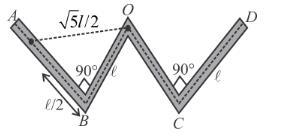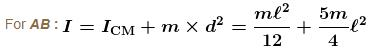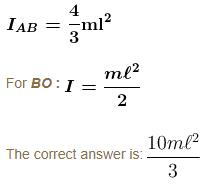Courses

# Rigid Body Dynamics MCQ Level - 2

## 10 Questions MCQ Test Basic Physics for IIT JAM | Rigid Body Dynamics MCQ Level - 2

Description
This mock test of Rigid Body Dynamics MCQ Level - 2 for Physics helps you for every Physics entrance exam. This contains 10 Multiple Choice Questions for Physics Rigid Body Dynamics MCQ Level - 2 (mcq) to study with solutions a complete question bank. The solved questions answers in this Rigid Body Dynamics MCQ Level - 2 quiz give you a good mix of easy questions and tough questions. Physics students definitely take this Rigid Body Dynamics MCQ Level - 2 exercise for a better result in the exam. You can find other Rigid Body Dynamics MCQ Level - 2 extra questions, long questions & short questions for Physics on EduRev as well by searching above.
QUESTION: 1

### A uniform equilateral prism of mass m rests on a rough horizontal surface with coefficient of friction mu. A horizontal force F is applied on the prism as shown in the figure. If the coefficient of friction is sufficiently high so that the prism does not slide before topping, then the minimum force required to topple the prism is :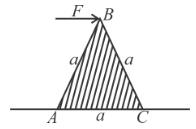Solution:

The tendency of rotation will be about the point C. For minimum force, the torque of F about C has to be equal to the torque of mg about C.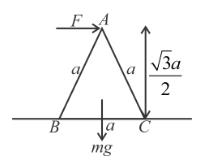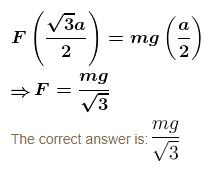QUESTION: 2

### Two points of a rod move with velocities  3v and v perpendicular to the rod and in the same direction, separated by a distance r. Then the angular velocity of the rod is :

Solution: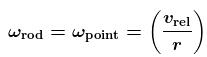vrel bring the velocity of one point w.r.t. other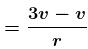and r being the distance between them

= 2/vr

QUESTION: 3

### Two identical uniform solid spherical balls A and B of mass m each are placed on a fixed wedge as shown in figure. Ball B is kept at rest and it is released just before two balls collides. Ball A rolls down without slipping on inclined plane and collide elastically with ball B. The kinetic energy of ball A just after the collision with ball B is :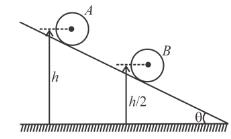Solution:

Just before collision between two balls

potential energy lost by Ball A = kinetic energy gained by Ball A.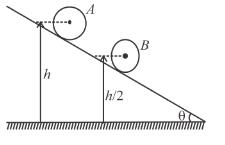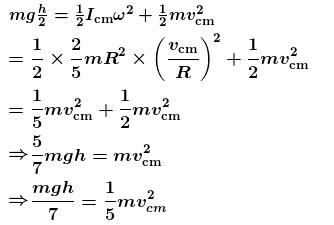After collision only translational kinetic energy is transferred to ball B

So just after collision rotational kinetic energy of Ball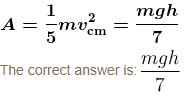QUESTION: 4

A uniform ring of radius R rolls without sliding. The radius of curvature of the path followed by any particle of the ring at the highest point of its path will be :

Solution: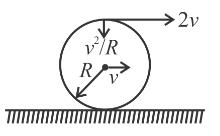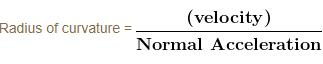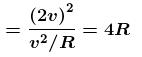QUESTION: 5

A uniform thin rod of mass m and length L is held horizontally by two vertical strings attached to the two ends. One of the string is cut. Find the angular acceleration soon after it is cut :

Solution:

Immediately after string connected to end B is cut, the rod has tendency to rotate about point A. Torque on rod AB about axis passing A and normal to plane of paper is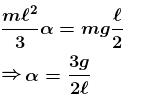Alternative

Applying Newton's law of centre of mass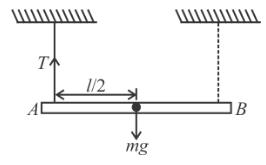mg – T = ma     ....(1)

Writing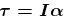about centre of mass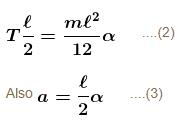From (1), (2) and (iii)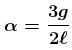QUESTION: 6

A uniform ring of radius R is given a back spin of angular velocity V0/2R and thrown on a horizontal rough surface with velocity of centre to be V0. The velocity of the centre of the ring when it starts pure rolling will be :

Solution:

Here u = v0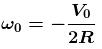At pure rolling :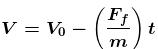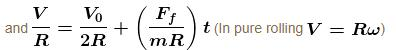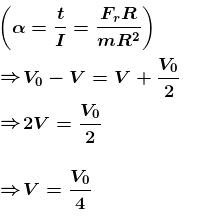QUESTION: 7

A uniform triangular plate ABC of mass m and moment of inertia I  (about an axis passing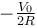through A and perpendicular to plane of the plate) can rotate freely in the vertical plane about point A as shown in figure. The plate is released from the position shown in the figure. Line AB is horizontal. The acceleration of centre of mass just after the release of plate is :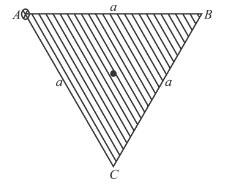Solution: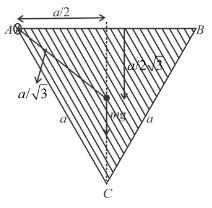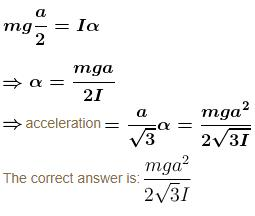QUESTION: 8

A uniform rod of mass M and length L lies radially on a disc rotating with angular speed ω in a horizontal plane about its axis. The rod does not slip on the disc and the centre of the rod is at a distance R from the centre of the disc. Then the kinetic energy of the rod is :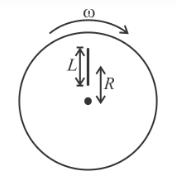Solution:

Moment of inertia of the rod about w.r.t. the axis through centre of the disc 1 to the plane line is : (by parallel axis theorem).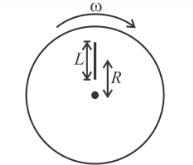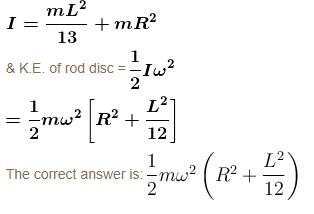QUESTION: 9

In the figure shown, a small solid spherical ball of mass m can move without sliding in a fixed semicircular track of radius R in vertical plane. It is released from the top. The resultant force on the ball at the lowest point of the track is :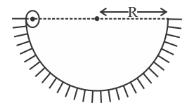Solution:

From conservation of energy, the kinetic energy of ball at lowest portion is (vc = speed of centre of ball)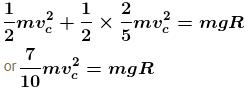Since net tangential force on sphere at lowest point is zero, net force on sphere at lowest position is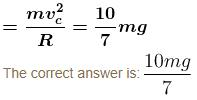QUESTION: 10

A uniform thin rod of length 4l, mass m is bent at the points as shown in the figure. What is the moment of inertia of the rod about the axis passing through point O and perpendicular to the plane of the paper.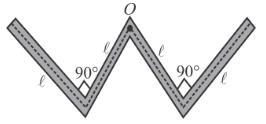Solution:

The given structure can be broken into 4 parts